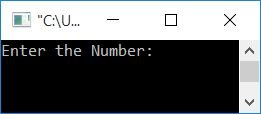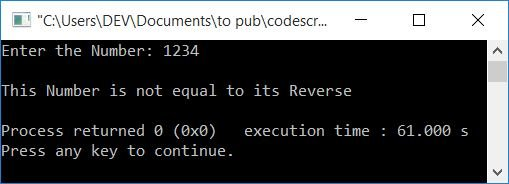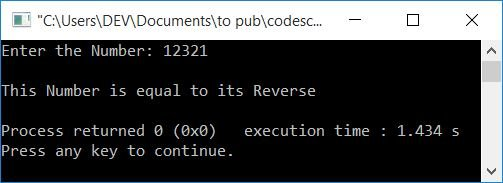# C++ Program to Determine Whether a Number Is Equal to Its Reverse

Here you will learn and get code for checking whether the input number is equal to its reverse or not in C++.

To check whether the original number is equal to its reverse or not in C++ programming, you have to ask from user to enter the number first. Then initialize its value (number entered by user) to a variable say orig and then reverse that number (entered by user). Finally, as shown in the following program, it compares its reverse to the original to determine whether the reverse is equal to the original or not.

```#include<iostream>
using namespace std;
int main()
{
int num, orig, rev=0, rem;
cout<<"Enter the Number: ";
cin>>num;
orig = num;
while(num>0)
{
rem = num%10;
rev = (rev*10)+rem;
num = num/10;
}
if(orig==rev)
cout<<"\nThis Number is equal to its Reverse";
else
cout<<"\nThis Number is not equal to its Reverse";
cout<<endl;
return 0;
}```

This program was built and runs under the Code::Blocks IDE. Here is its sample run:NNow supply any input as input, say 1234, to check whether its reverse is equal to the number itself or not, as shown in the output given below:Here is another sample run with user input as 12321:Note: Make sure to initialize the input value to a variable, say orig, before reversing the number.

As you can see from the above program, we have reversed the number and then compared it with the original (the value stored in orig).

#### The same program in different languages

C++ Quiz

« Previous Program Next Program »